Courses
Courses for Kids
Free study material
Free LIVE classes
More

# One Digit DivisionLIVE
Join Vedantu’s FREE Mastercalss

## Introduction

Division is the inverse mathematical action of multiplication. We divide a number into equal parts when we divide it. Division is the process of repeatedly subtracting integers. Let us look at a real-life example to learn more about this. Assume you need to give 30 candies among 3 of your buddies in an equitable manner. To ensure that each child receives an equal quantity of candy, divide 30 by the number of friends, which is 3. This is how division functions.

## Division By 1 Digit Number

A division equation can be expressed in two ways: sentence form and lengthy division form. Basic arithmetic procedures are employed in the long-division approach. The dividend is the number that is split into equal groups in this case. The divisor is the number of groups by which the dividend is split, and the quotient is the result of the division.

The remainder is a significant portion of a division equation since it is the part that remains after the division operation is completed. The remainder in the preceding case is zero since there is no integer left over.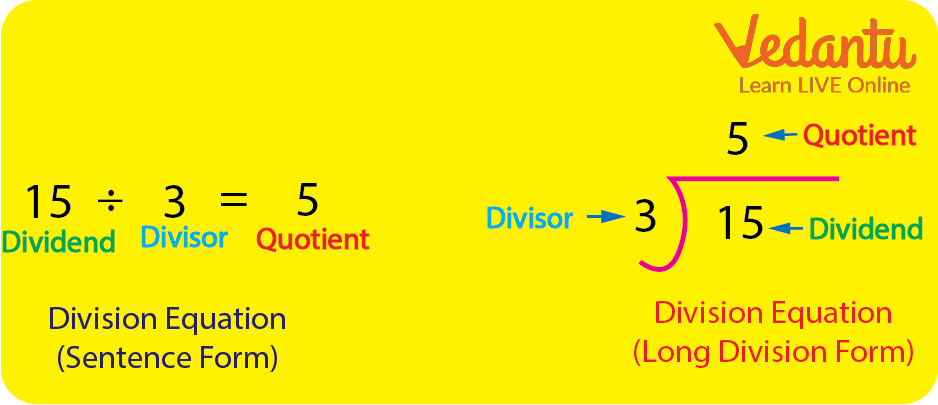Example of Division 2-Digit By 1 Digit

## One Digit Division Sums

Let us take another example of division 2-digit by 1-digit to understand it better.

Example 1: Let's divide 65 by 5.

Step One: The first digit 6 is greater than the divisor (5). So we can directly use 5 in this case and subtract 5 from 6. This will give us the remainder as 1 and the quotient as 1.

Step Two: Bring down the next digit and form a new number with the remainder. The new number is 15.

Step Three: 15 is directly divisible by 5 as 5 multiplied by 3 is 15. After their subtraction, we will get the remainder as 0 and the new quotient will be 13.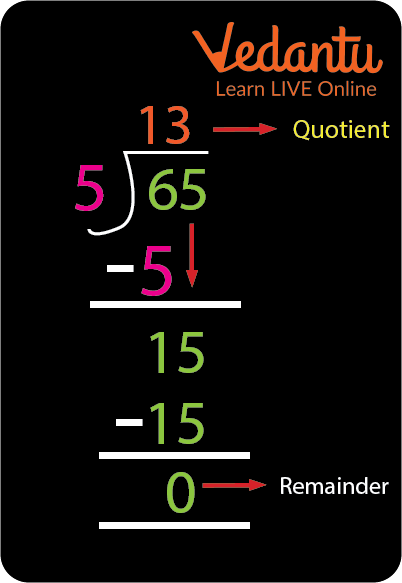Example of Division of 2 Digit Number By 1 Digit

Example 2: Divide 2782 by 3.

Step One: The first digit is less than the divisor which will not be divisible by 3 so we need to take the next digit. 27 is divisible by 3. So we subtract 27 by 27 and get the remainder as 0 and the quotient as 9.

Step Two: Let's take the next digit down. 8 is now subtracted by 6 which leaves us with the remainder of 2 and the new quotient becomes 92.

Step Three: The next digit 2 is now brought down and the new number becomes 22. This can be subtracted from 21 which comes in table 3. We get the remainder as 1. And the quotient is 927.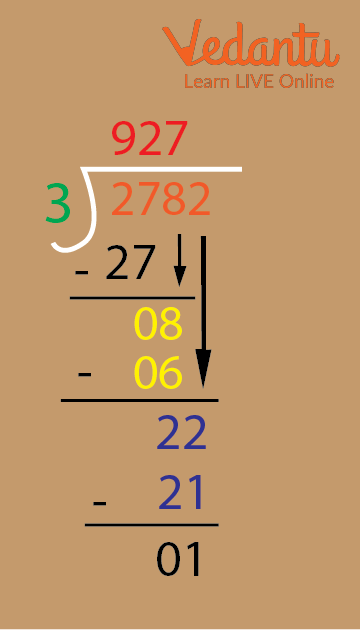Division Example

## Conclusion

The one-digit division is the easiest type of division. The one-digit divisor can range from 1 to 9. The basis of the division is to find multiple, subtract, bring down the next digit and repeat. A one-digit division is much easier than a two and three-digit division.

## Sample Questions

1. If there are 55 pencils to be distributed among 5 people. How many pencils would each one get?

a. 30

b. 40

c. 3

d. 11

Ans: 11

Explanation: Step one: Find the multiple of the first digit and subtract it from that.

Step two: Bring down the next digit and find the multiple of the new number formed.

Step three: The remainder comes out to be 0.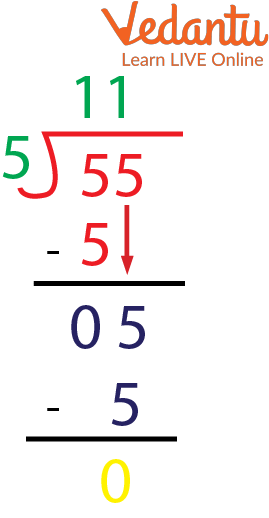Division of 55 By 5

2. Divide 120 by 4.

a. 30

b. 40

c. 3

d. 10

Ans: 30

Explanation: As 4 when multiplied by 3 gives us 12 so 12 is divisible by 4. This will give us a remainder of 0. As the next digit is also 0 the new number is 0 and 4 when multiplied by 0 will give us 0. Making the quotient 30.

3. We need to distribute 369 candies among 9 students. Each student will receive.

a. 40

b. 41

c. 42

d. 43

Ans: 41

Explanation: total number of candies is 369.

The number of students is 9.

Each student will get 41 candies.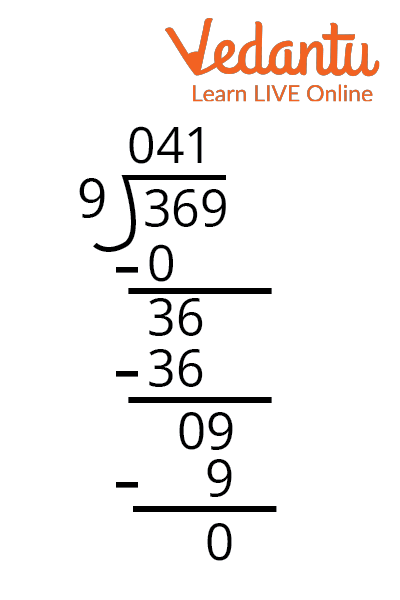Division of 369 By 9

Last updated date: 23rd Sep 2023
Total views: 88.8k
Views today: 1.88k

## FAQs on One Digit Division

1. Under what circumstances may the quotient contain a zero?

Ans: A quotient may contain a zero when the divisor is greater than the dividend. In such cases the quotient would have a zero and then the next digit of the dividend can be brought down for further process of division.

2. How can you divide a bigger whole number by a lower whole number using place values?

Ans: To divide a bigger number by a smaller number we need to align the integers vertically and compare them to find how the division will take place. To perform long division it is necessary to have the smaller number as the divisor and the larger number as the dividend.

3. What is the difference between division and multiplication?

Ans: The difference between division and multiplication is that in division we bring down the value to the lowest possible number and in multiplication, we add on to the value to bring it to the highest possible value.

4. What are the different types of division?

Ans: The division is divided into two parts: partitive models and quotative models. When splitting a number into a known number of slots, the partitive is employed. For example, dividing 4 into two slots allows us to determine how many objects will be in each slot. When splitting a number into slots of a measurable quantity, quotative division is utilized. For example, dividing 4 into slots of 2 allows us to calculate how many slots may be constructed.

5. What are the three divisional parts?

Ans: The three basic and important parts of the division are dividend, quotient, and divisor where the divisor is always smaller than the dividend.